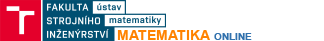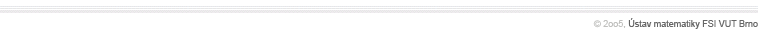CurvesPlanar curves, 3D-curves, smooth regular curves, directing an open curve, directing a closed curve, parametric definition of a curve, rectifiable curves, length of a regular curve, astroid, cycloid, cardoid, clothoid, folium of Descartes, helixLine integral - basic methodsLine integral in scalar fields, definition, basic properties and methods of calculation in E2 and E3, line integral in vector fields, definition, basic properties and methods of calculation in E2 and E3,Line integral - advanced methodsLine integrals in conservative vector fields, potential of a vector field, calculating the potential in E2 and E3, nabla operator, curl of a vector field, Green's theorem and its applications